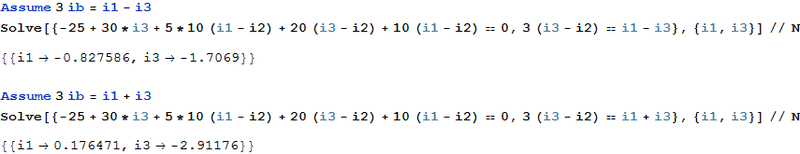# Arbitrary current with mesh analysis

The Electrician
Gold Member
Something goes wrong in your last calculation The Electrician. See post no.17 for i3 and ib values.

I missed the minus sign on i3 in post #17. You had i3=-2.9117647 A.
I think it helps to leave spaces in appropriate places like this: i3 = -2.9117647 A

So I'll redo the calculation:

Check -25+30*i3+5*ua+20*ib+ua, has to be 0.

OK, let's evaluate all the terms using your values for i1, i2 and i3, using the fact that ua = 10*(i1-i2), ib = i3-i2
Listing the results, I get:

-25 = -25
30*i3 = -87.352941
5*ua = 108.82353
20*ib = -18.235294
ua = 21.764706

These add up to .000001, essentially zero.

For any value of i1, a value of i3 = -27/10 - (6*i1)/5 gives a value of zero. However, this is only the solution to one equation, and not a solution to the complete network. It is also necessary to satisfy the equation 3ib = i1-i3, but you assumed i1=3*ib-i3, which is incorrect.

The Electrician
Gold Member
Here are two solutions:Unfortunately, the second one is incorrect.

It has to be a conventional rule in order to state if i1=3ib-i3 or 3ib=i1-i3
since i1 and i3 are virtual [ghosts] but 3ib it is a concrete one.
Since I am only an engineer –master it does not help here, I think-and not a professor
I get the professors’ opinion.The Electrician
Gold Member
It has to be a conventional rule in order to state if i1=3ib-i3 or 3ib=i1-i3
since i1 and i3 are virtual [ghosts] but 3ib it is a concrete one.
Since I am only an engineer –master it does not help here, I think-and not a professor
I get the professors’ opinion.The branch current 3ib is clearly composed of some combination of the loop currents i1 and i3.

The loop current i1 is in the same direction as the 3ib source current, and i3 is in the opposite direction, thus 3ib = i1 - i3.

Post #23 indicates that simulation gives the same result as assuming 3ib = i1 - i3.